Compare Mean and Median in Less Than 20 Seconds:

by on February 2nd, 2013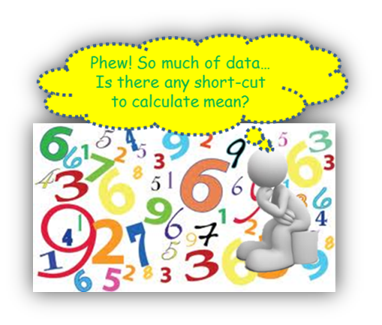Purpose

In a table analysis question that contains a long list, calculating mean through traditional method takes time.

To save time, we will introduce two techniques that will allow you to logically deduce whether the Mean is greater than or less than the median.

To illustrate my point, let’s review question #24 from OG13 for discussion.

The table lists data on the 22 earthquakes of magnitude 7 or greater on the Richter Scale during a recent year. Times are given in hours, minutes, and seconds on the 24-hour Greenwich Mean Time (GMT) clock and correspond to standard time at Greenwich, United Kingdom (UK). Latitude, measured in degrees, is 0 at the equator, increases from 0 to 90 proceeding northward to the North Pole, and decreases from 0 to –90 proceeding southward to the South Pole. Longitude, also measured in degrees, is 0 at Greenwich, UK, increases from 0 to 180 from west to east in the Eastern Hemisphere, and decreases from 0 to –180 from east to west in the Western Hemisphere.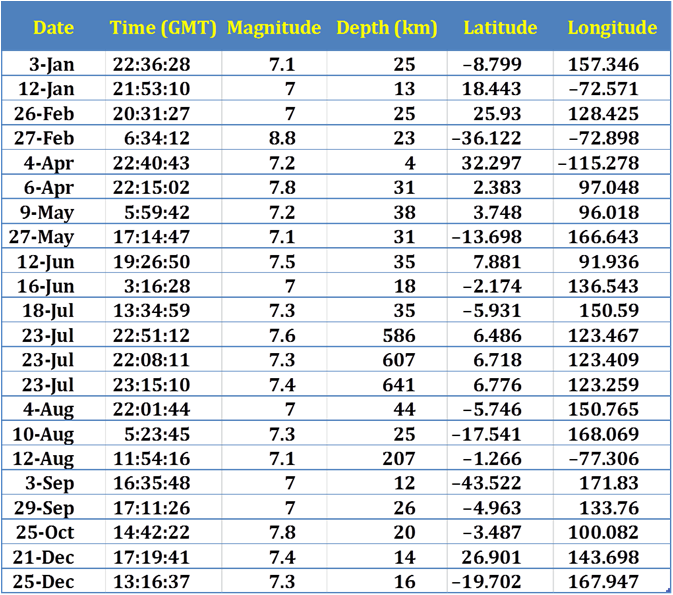For each of the following statements, select Yes if the statement is true based on the information provided; otherwise select No.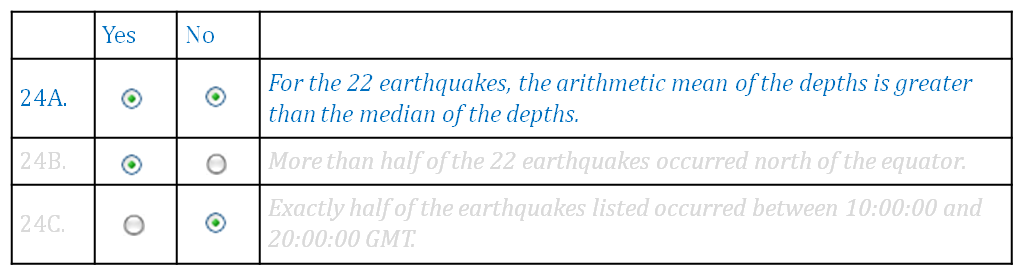The focus of our discussion will be question # 24A.

Standard approach

“For the 22 earthquakes; the arithmetic mean of the depths is greater than the median of the depths.”

In mathematical terms: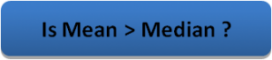The question asks us to compare mean and median. Our natural instinct will be to first calculate the mean and then the median.

Mean –> The Mean involves handling 22 data points. Summing up all 22 data points and dividing the sum by 22 is quite time consuming. The mean comes out to be 112.56.

Median –>

1. Median is the value of the middle-most cell of depth column, when data points are arranged in ascending order.
2. In this dataset, there are 22 elements.
3. So middle most value = mean of 11th and 12th values
4. Median = 25.5

Now let’s review the two approaches.

Approach 1 – “Limited set observation”

Per the approach, we will at first calculate median, since median is less calculation intensive as compared to mean.

Median = 25.5 (Following the same approach as in “standard approach”)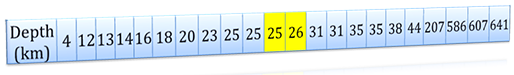Mean –

Fundamental principle – In a series of all positive numbers, The Mean of the series is always greater than the sum of limited data divided by total # of data points in the setWhen we glance at the dataset, we find that the last value 641 is disproportionately high. This implies that mean of the all depths must be greater than 1/22 times 641. This equals to 1/22*(641) = 29.136 km. Now, 29.136 km itself is already greater than the median depth (25.5 km), so the actual mean of the depths must be greater than the median of the depths. So we could arrive at the answer without actually calculating the exact mean of the list.

This approach has also been used by OG.

Approach 2: The Sherlock Method – “Logical deduction by observation”

This approach is along the lines of the Approach 1- Limited set Observation approach, but there is no calculation involved. In fact this approach relies on data observation only.

Median- Instead of exactly calculating the median, we will make a few observations. After all to find the exact median, we did have to go through some effort (as shown in standard approach). We know that median is the middle most value.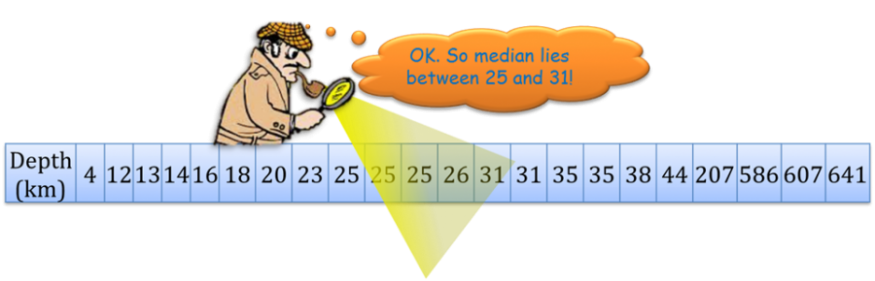Just observing this data set, we can be confident that the median will fall between 25 and 31.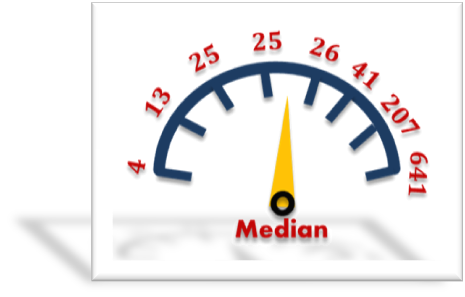Mean- Let us understand a property of mean in relation to ascending order listed dataset.

The given data set of depth is arranged in ascending order, & bottom cell values are disproportionately higher than other cell values. As all the data points lay equal importance for mean, this implies that mean will drift towards heavy values.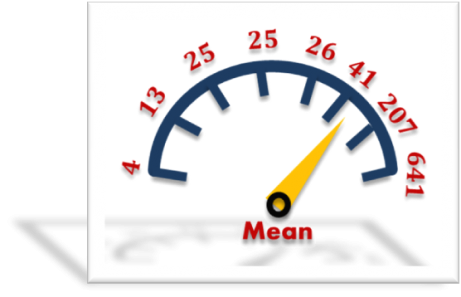So it is obvious that mean value will be more than the median. In fact it will be much higher than the median value in this specific question.

Just to recollect the question, “For the 22 earthquakes; the arithmetic mean of the depths is greater than the median of the depths.” So the answer to the question is YES.

The best thing about this approach is that we don’t need to do any calculation. Mere observation of data & use of basic concept is enough to determine the answer.

Table given below presents a few salient features of mean and median.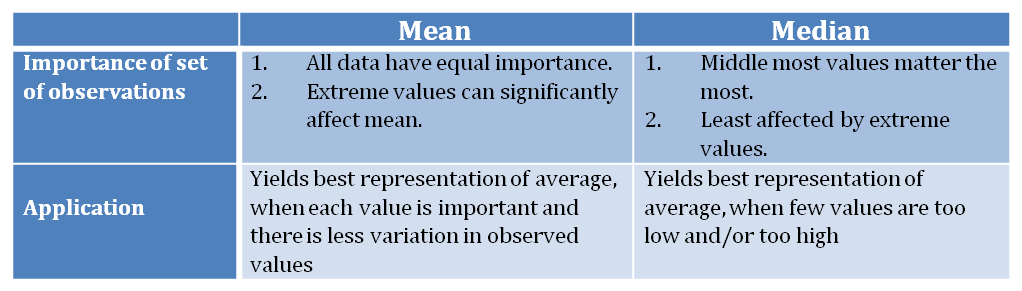Another scenario

Few top cell values are disproportionately low and bottom cell values are disproportionately high.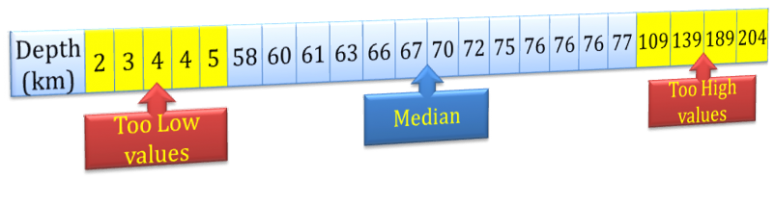In this scenario, we cannot infer that the mean is less or more than the median. The top 5 cells’ values will drift the mean towards a lower value, whereas the bottom 4 cells’ values will drift it towards a higher value. So it is difficult to infer by mere observation the way in which the mean will drift more.

So what to do in this scenario?

Well, GMAT is not going to test exactly this kind of scenario. Had this scenario been presented to you in the exam, there would have been some other concept applicable to deduce the answer. GMAT lays more importance on application of concepts than on long calculations.

Key Take-Aways:

When comparing mean and median, first arrange the data in ascending order:

• If values in the bottom cells are too high, then Mean > Median;
• If values in the top cells are too low and bottom cells are too high, then do not apply this approach.

Practice Question

13 students from a school were rated for their proficiency in 4 sports – higher the rating, more the proficiency. Table Tennis and Basketball scores are out of 100 points, Lawn Tennis out of 20 points, and badminton out of 50 points.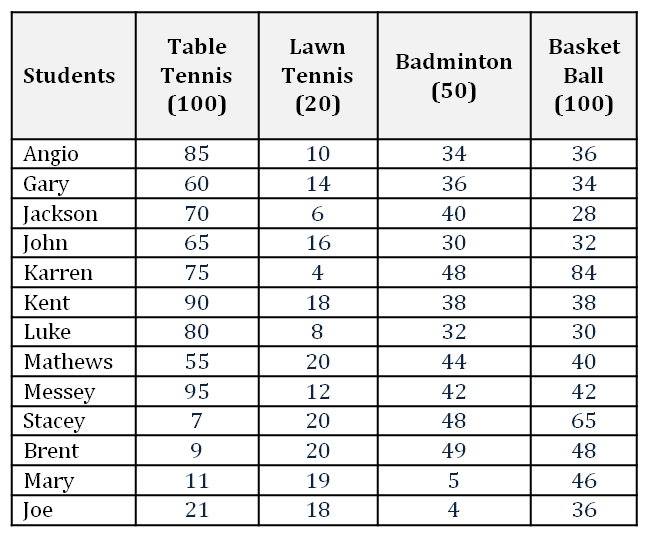For each of the following statements, select ‘Yes’ if statement is true based solely on the information provided in the table; otherwise select ‘No’.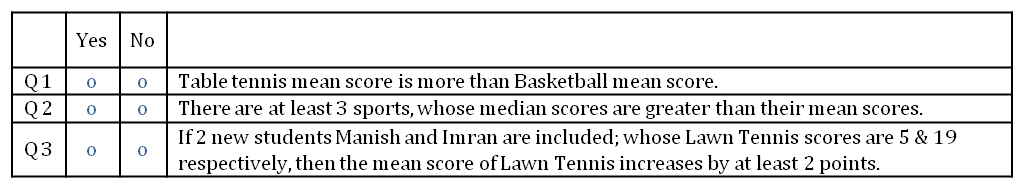Enjoy averaging,

Shalabh

•Q1, Q2, Q3 -> Yes
Q3 is quite simple, as (5+19)/2 is 12>2.

••